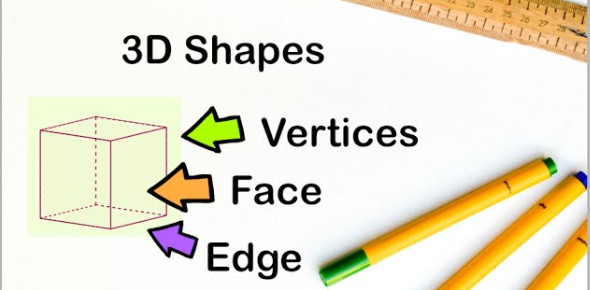# 3D Shapes Faces, Vertices And Edges Quiz

10 Questions | Total Attempts: 5472Settings3D shapes have faces, vertices and edges. These features differentiate one shape from the other. Having covered 3d shapes in the math class this week you need to polish up on what you know so far. Give this revision quiz a try and ensure to read up on the questions you fail.

Questions and Answers
• 1.
Compare the three-dimensional solids below. Which statement below is true?
• A.

The triangular prism has more edges than the cube.

• B.

The cube and the triangular prism have the same number of vertices.

• C.

The cube has more edges than the triangular prism.

• 2.
Which two solids below have the same number of faces?
• A.

Solids B and C

• B.

Solids A and B

• C.

Solids A and D

• 3.
Which shape has two faces?
• A.

B

• B.

C

• C.

D

• 4.
Which shape is a cube?
• A.

A

• B.

C

• C.

D

• 5.
Which statement below is true?
• A.

This solid figure had 6 corners (vertices).

• B.

This solid figure is called a pyramid.

• C.

This solid figure has 8 faces.

• 6.
How many faces does this shape have?
• A.

4

• B.

5

• C.

6

• 7.
Faces ______________
• 8.
Edges _____________
• 9.
Vertices _____________
• 10.
Write what you know about this 3d shape.
Related Topics